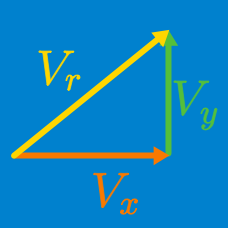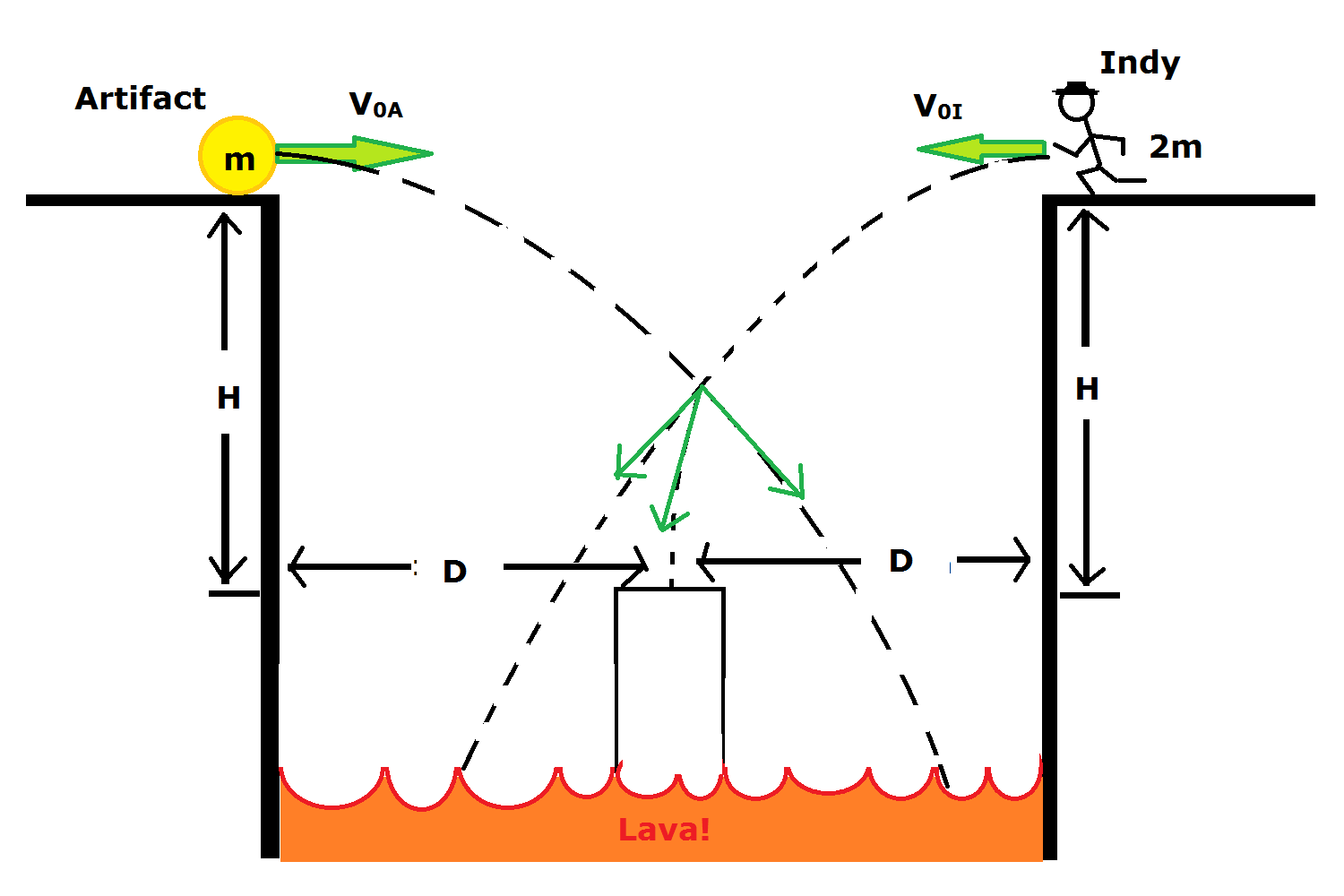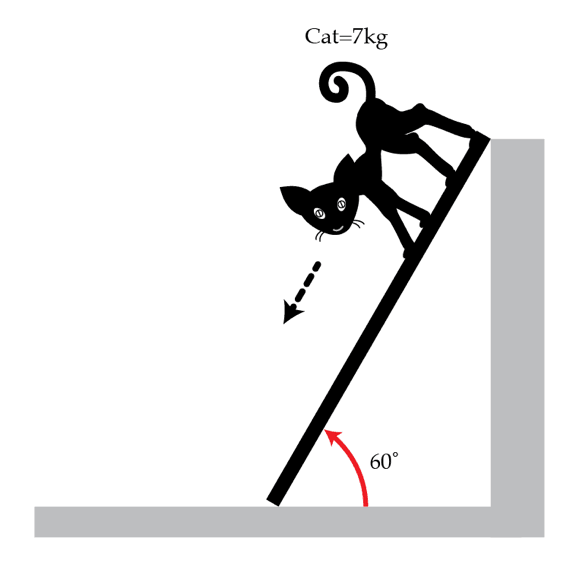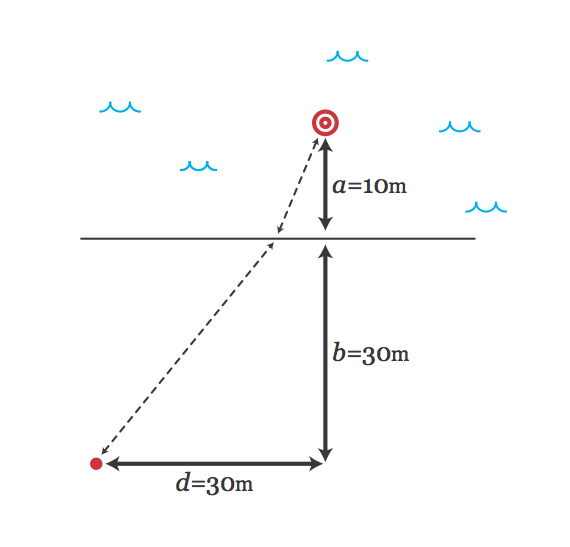Classical Mechanics

# Vector Kinematics: Level 4-5 ChallengesAn ancient artifact is thrown horizontally off of a ledge overlooking a pool of lava. It has an initial speed of $v_{0A}$ and has mass $m$. There is a raised platform in the middle of the lava pool, at a distance $D$ to the left of the ledge, and $H$ below the ledge. The artifact is going to overshoot the platform.

Indiana Jones is standing on a ledge opposite the first ledge, at the same distance from the platform and the same elevation as the first ledge. He has mass $2m$. He takes a running horizontal leap off his ledge at the same instant the artifact is thrown.

If $v_{0A} = 20.2 \frac{m}{s}$, $D = 15.3 m$, and $H = 10.1 m$, how fast [$v_{0I}$] must Indy leap, so that he grabs the artifact and lands safely on the platform? Give your answer in $\frac{m}{s}$, rounded to 3 significant figures.

For acceleration due to gravity, use $g = 9.81 \frac{m}{s^{2}}$.

A cat of mass $M=7~\text{kg}$ decides to descend down a light inclined plank of length $L=6~\mbox{m}$ which makes an angle of $\alpha=60^{\circ}$ with the horizontal, as shown in the figure below. The friction between the plank and the surfaces (the wall and the floor) can be neglected. The cat knows that walking on the plank may be dangerous. Nevertheless, the cat figures out a way to descend so that the plank remains at rest at all times. How long in seconds does it take the cat to reach the floor? The initial velocity of the cat is zero. You may ignore the mass of the plank. The cat remains in contact with the plank until he reaches the floor.Details and assumptions

$g=9.8~\text{m}/\text{s}^2$

Margo and Sven are outside in a field, standing right next to each other and talking. We'll put x,y coordinates on the field and measure everything in meters. Ursula, a friend of both Margo and Sven, is also outside walking due north (positive y-direction) at $1~\mbox{m/s}$. When Ursula crosses the origin (at $t=0$) Margo and Sven (at coordinates $(100,0)$) notice her and decide to walk over and say hello.

Margo knows a little physics, and so she figures out how she should walk at $2~\mbox{m/s}$ to meet Ursula in the shortest amount of time. Sven doesn't know any physics, so he just always walks directly towards Ursula, also at $2~\mbox{m/s}$, until he catches up to her.

If the time Sven meets Ursula is $t_s$ and the time Margo meets Ursula is $t_m$, what is $t_s-t_m$ in seconds?

A family decides to go to the lake on their summer vacation. Their child is small, just around 4 or 5 years old and is very excited. When they get to the lake, the child runs immediately into the water while his parents set up their blanket on a little beach by the lake. Out in the lake, the child steps on a rock, hurts his toe, and begins to cry for his mom to come get him.Mom's velocity is $v_s=3~m/s$ on the sand and $v_w=1~m/s$ in the water. The distance between the mom and the child is given in the figure, with $d=30~m$, $a=10~m$ and $b=30~m$. What's the minimum time in seconds for her to reach her child?

Details and assumptions

• Remember, the quickest path between 2 points on a plane is a straight line (if you travel at constant velocity).
• There are a couple ways to do this problem, but one straightforward way involves solving a quartic equation, which you can do here.

A chicken decides to cross a road. The road is perfectly straight, runs north-south, and is full of cars driving by from south to north. The cars have a log-normal distribution for the distance between each car. However, the cars all travel at 80 km/hr.

The chicken stands next to the road on the west side, waits until a car goes by, and then immediately starts to cross the road at 0.25 m/s. What angle should the chicken cross at to maximize her chance of making it across the road without getting hit by a car? Express your answer as radians north of east.

Details and assumptions

• The chicken is a point particle.
• Assume for simplicity that a car is the same width as the road, so that if the chicken is on the road when the car reaches her, she gets hit.
×